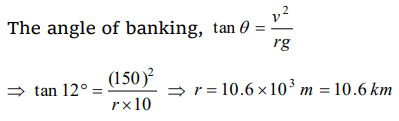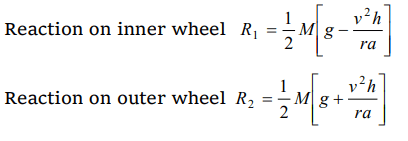## Motion in a Plane Questions and Answers Part-3

1.Two bodies of mass 10 kg and 5 kg moving in concentric orbits of radii R and r such that their periods are the same. Then the ratio between their centripetal acceleration is
a) R / r
b) r / R
c) $R^{2}\diagup r^{2}$
d) $r^{2}\diagup R^{2}$

Explanation:2. The ratio of angular speeds of minute hand and hour hand of a watch is
a) 1 : 12
b) 6 : 1
c) 12 : 1
d) 1 : 6

Explanation:3. A car travels north with a uniform velocity. It goes over a piece of mud which sticks to the tyre. The particles of the mud, as it leaves the ground are thrown
a) Vertically upwards
b) Vertically inwards
c) Towards north
d) Towards south

Explanation: The particle performing circular motion flies off tangentially

4. An aircraft executes a horizontal loop with a speed of 150 m/s with its, wings banked at an angle of $12^{\circ}$ . The radius of the loop is $\left(g=10m\diagup s^{2}\right)$
a) 10.6 km
b) 9.6 km
c) 7.4 km
d) 5.8 km

Explanation:5. A particle is moving in a horizontal circle with constant speed. It has constant
a) Velocity
b) Acceleration
c) Kinetic energy
d) Displacement

Explanation:6. A motor cyclist moving with a velocity of 72 km/hour on a flat road takes a turn on the road at a point where the radius of curvature of the road is 20 meters. The acceleration due to gravity is 10 $m\diagup s^{2}$. In order to avoid skidding, he must not bend with respect to the vertical plane by an angle greater than
a) $\theta=\tan^{-1}6$
b) $\theta=\tan^{-1}2$
c) $\theta=\tan^{-1}25.92$
d) $\theta=\tan^{-1}4$

Explanation:7. A train is moving towards north. At one place it turns towards north-east, here we observe that
a) The radius of curvature of outer rail will be greater than that of the inner rail
b) The radius of the inner rail will be greater than that of the outer rail
c) The radius of curvature of one of the rails will be greater
d) The radius of curvature of the outer and inner rails will be the same

Explanation: The radius of curvature of outer rail will be greater than that of the inner rail

8. The angular speed of a fly wheel making 120 revolutions/minute is
a) $2\pi rad\diagup s$
b) $4\pi^{2} rad\diagup s$
c) $\pi rad\diagup s$
d) $4\pi rad\diagup s$

Explanation:9. A particle is moving on a circular path with constant speed, then its acceleration will be
a) Zero
d) Constant acceleration

10. A car is moving on a circular path and takes a turn. If $R_{1} and R_{2}$  be the reactions on the inner and outer wheels respectively, then
a) $R_{1} = R_{2}$
b) $R_{1} < R_{2}$
c) $R_{1} > R_{2}$
d) $R_{1} \geq R_{2}$